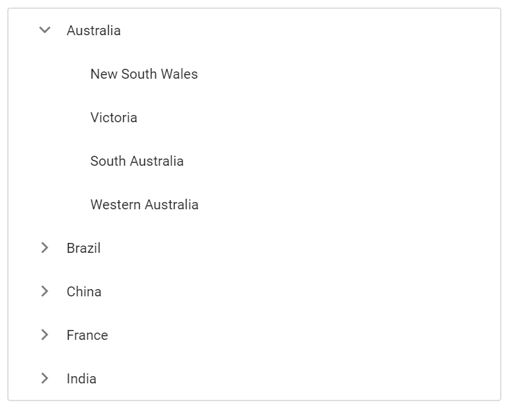Search results

Getting started with ASP.NET MVC TreeView control

18 Jan 2022 / 1 minute to read

This section briefly explains about how to include a simple ASP.NET MVC TreeView in your ASP.NET MVC application. You can refer ASP.NET MVC Getting Started documentation page for introduction part part of the system requirements and configure the common specifications.

TreeView control can be rendered by using the EJS().TreeView() tag helper in ASP.NET MVC application. Add the below simple code to your index.cshtml page which is available within the Views/Home folder, to initialize the TreeView.

The following example shows a basic TreeView control.

razor
gettingstarted.cs
@Html.EJS().TreeView("listdata").Render();
public IActionResult Default()
{
return view();
}

Binding data source

TreeView can load data either from local data sources or remote data services. This can be done using the dataSource property that is a member of the fields property. The dataSource property supports array of JavaScript objects and DataManager. Here, an array of JSON values is passed to the TreeView control.

razor
selfreferential.cs
@Html.EJS().TreeView("listdata").Fields(field=>
field.Id("id").ParentID("pid").Selected("selected").
Expanded("expanded").Text("name").HasChildren("hasChild")
.DataSource(ViewBag.dataSource)).Render()
public IActionResult LocalData()
{

List<object> listdata = new List<object>();
{
id = 1,
name = "Australia",
hasChild = true,
expanded = true
});
{
id = 2,
pid = 1,
name = "New South Wales",

});
{
id = 3,
pid = 1,
name = "Victoria"
});

{
id = 4,
pid = 1,
name = "South Australia"
});
{
id = 6,
pid = 1,
name = "Western Australia",

});
{
id = 7,
name = "Brazil",
hasChild = true
});
{
id = 8,
pid = 7,
name = "Paraná"
});
{
id = 9,
pid = 7,
name = "Ceará"
});
{
id = 10,
pid = 7,
name = "Acre"
});
{
id = 11,
name = "China",
hasChild = true
});
{
id = 12,
pid = 11,
name = "Guangzhou"
});
{
id = 13,
pid = 11,
name = "Shanghai"
});
{
id = 14,
pid = 11,
name = "Beijing"
});
{
id = 15,
pid = 11,
name = "Shantou"

});
{
id = 16,
name = "France",
hasChild = true

});
{
id = 17,
pid = 16,
name = "Pays de la Loire"

});
{
id = 18,
pid = 16,
name = "Aquitaine"

});
{
id = 19,
pid = 16,
name = "Brittany"

});
{
id = 20,
pid = 16,
name = "Lorraine"
});
{
id = 21,
name = "India",
hasChild = true

});
{
id = 22,
pid = 21,
name = "Assam"

});
{
id = 23,
pid = 21,
name = "Bihar"
});
{
id = 24,
pid = 21,